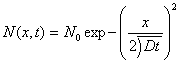# Limited-Source Diffusion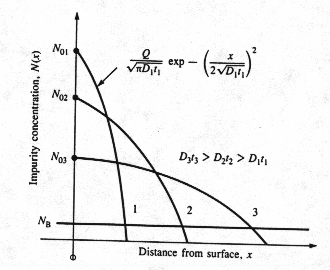Fig. 3 A gaussian distribution results from a limited-source diffusion or constant total dopant diffusion. As the Dt product increases, the diffusion front moves more deeply into the wafer and the surface concentration decreases. The area under each of the three curves is the same. 

Limited-source diffusion is modeled mathematically using an impulse function at the silicon surface as the initial boundary condition. The magnitude of the impulse is equal to the dose Q.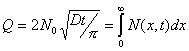Initial condition:

At t=0, N (x, 0)=0

Boundary Conditions: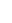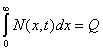And C (¥, t) = 0

The solution of Fick's second law that satisfies the initial and boundary conditions is given by: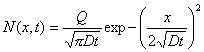By setting x = 0 we obtain the surface concentration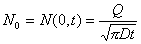The solution can be rewritten as